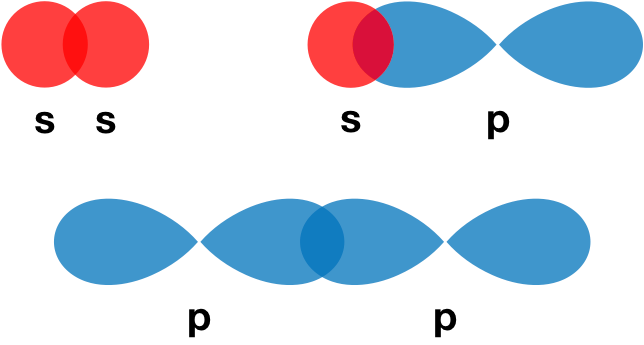# Problem: Indicate which orbitals overlap to form the sigma bonds in:a. BeBr2b. HgCl2c. ICN

###### FREE Expert Solution
95% (133 ratings)
###### FREE Expert Solution

We’re being asked to determine the orbitals that overlap to form sigma bonds in BeBr2, HgCl2, ICN.

Recall that σ (sigma) bonds are the single bonds in a molecule. They are formed from the end-on overlap of orbitals.For this problem, we need to do the following steps:

Step 1: Determine the central atom in the molecule.

Step 2: Calculate the total number of valence electrons present.

Step 3: Draw the Lewis structure for the molecule.

Step 4: Determine the hybridization of the central atom.

Step 5: Determine the orbitals that overlap to form the sigma bonds.

95% (133 ratings)###### Problem Details

Indicate which orbitals overlap to form the sigma bonds in:

a. BeBr2

b. HgCl2

c. ICN

What scientific concept do you need to know in order to solve this problem?

Our tutors have indicated that to solve this problem you will need to apply the Valence Shell Electron Pair Repulsion Theory concept. You can view video lessons to learn Valence Shell Electron Pair Repulsion Theory. Or if you need more Valence Shell Electron Pair Repulsion Theory practice, you can also practice Valence Shell Electron Pair Repulsion Theory practice problems.

What is the difficulty of this problem?

Our tutors rated the difficulty ofIndicate which orbitals overlap to form the sigma bonds in:a...as high difficulty.

How long does this problem take to solve?

Our expert Chemistry tutor, Dasha took 5 minutes and 5 seconds to solve this problem. You can follow their steps in the video explanation above.

What professor is this problem relevant for?

Based on our data, we think this problem is relevant for Professor Jones, Auzanneau & deLaat's class at University of Guelph.